# Architectural 100G Dual-Summing-Node-DFE PAM4 SerDes Receiver Model

This example demonstrates the use of an architecturally representative 100G dual-summing-node-DFE PAM4 SerDes receiver model using the library blocks from the SerDes Toolbox™ library and custom blocks. The more representative data path allows for a more accurate modeling of the adaptation of the clock phase, DFE tap weights and PAM4 threshold voltages. This example shows the necessity of managing the adaptive loop update factors to ensure that the numerous adaptive loops don't fight against each other.

### Example Overview

Historically, SerDes have been designed using a single summing node for DFE tap feedback. Due to decreasing design margins, the increasing difficulty in meeting timing requirements, and difficulty of routing high-speed clocks, this architectural topology has fundamental limits. An alternative topology uses two summing nodes, rather than one, each to process every other incoming symbol to ease timing margins and to reduce clock speeds at the expense of circuit complexity and area . This example of architecturally representative dual-summing-node-DFE PAM4 receiver model provides access to realize differences between summing node offsets, bandwidths and timing. The use of modular blocks for the DFE, adaptation engine, phase detector, loop filter and VCO allows for the collaboration between multiple teams and with the advantages of utilizing block interfaces for correlation and verification activities. The representative data path allows for a representative adaptation engine model and the exploration between interactions of the adaptation loops for the clock phase, DFE tap weights and PAM4 threshold voltages.

### Model Overview

Open the model by opening `DualSummingNodeSerdes.slx` file.The receiver (Rx) model contains the same analog front end, CTLE, VGA and memory-less non-linearity blocks, as the COM reference receiver detailed in the ADC IBIS-AMI Model Based on COM example and Architectural 112G PAM4 ADC-Based SerDes Model example.The last block in the receiver contains the dual-summing-node DFE and the clock recovery units.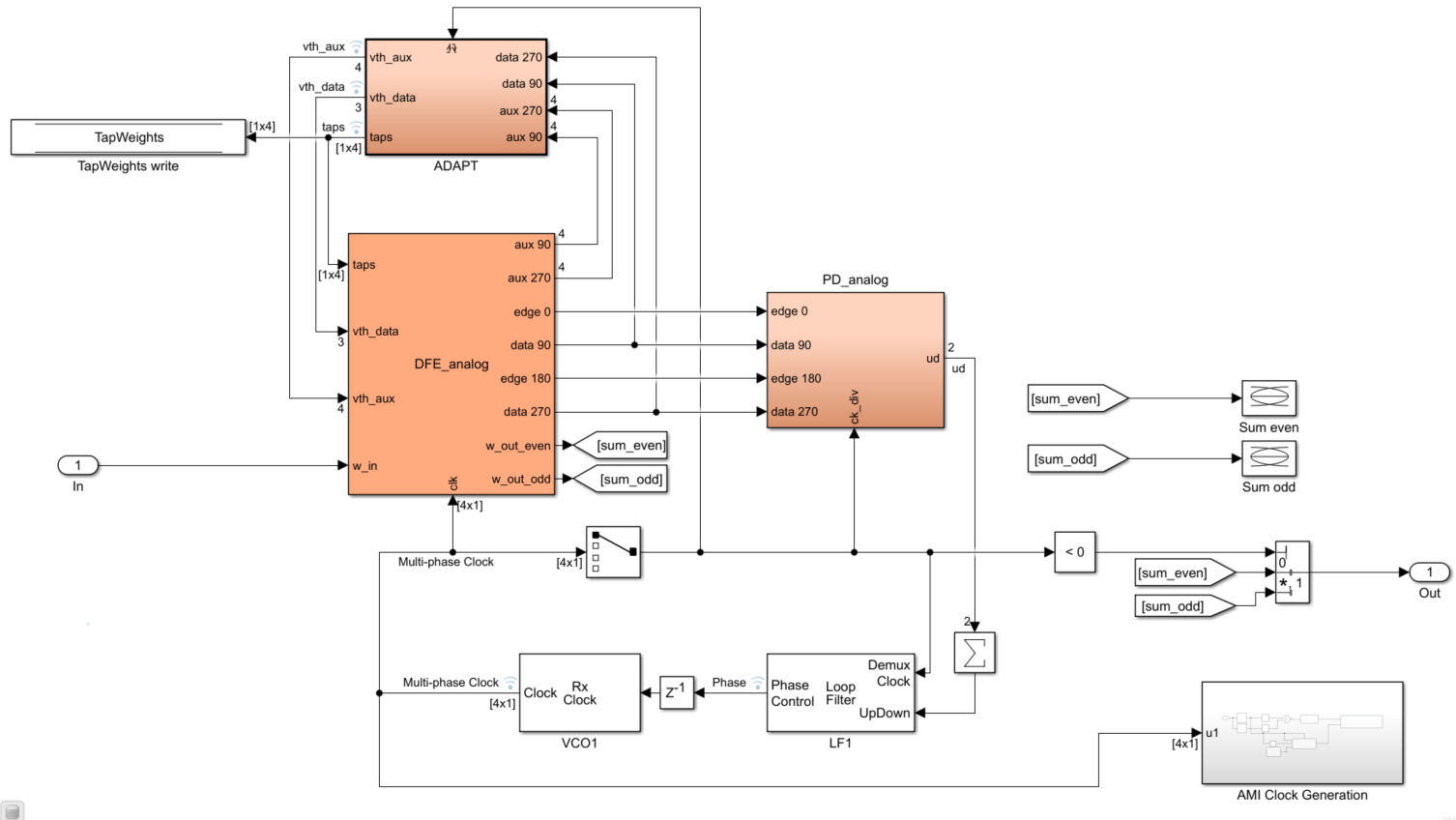### Dual Summing Node Description

The dual summing node DFE architecture relaxes the timing requirements by breaking the data path into two branches ("odd" and "even" in this example) that sample and apply digital-feedback-equalization (DFE) to every other data symbol. The partially equalized input signal, w_in, is fed to the even and odd summing nodes. The previous data decisions are appropriately weighted by the DFE tap weights and subtracted from the input signal to generate the even and odd summing node outputs, "w_out_even" and "w_out_odd", to further equalize the signal.The odd and even branches have their own set of PAM4 data samplers, auxiliary samplers (used to determine the PAM4 data sampler threshold voltages and DFE tap adaptation) and edge samplers (used in the Bang-Bang phase detection and clock recovery). These binary samplers can determine whether the input signal is above or below the sampler's threshold voltage. The auxiliary samplers levels (AUX +1, AUX +1/3, AUX -1/3 and AUX -1) or signal constellation levels will adapt to the observed signal constellation voltages depending on the observed samples. The ideal odd (yellow and red X's) and even (green and black X's) sampler positions (in time and voltage) of a PAM4 eye diagram are: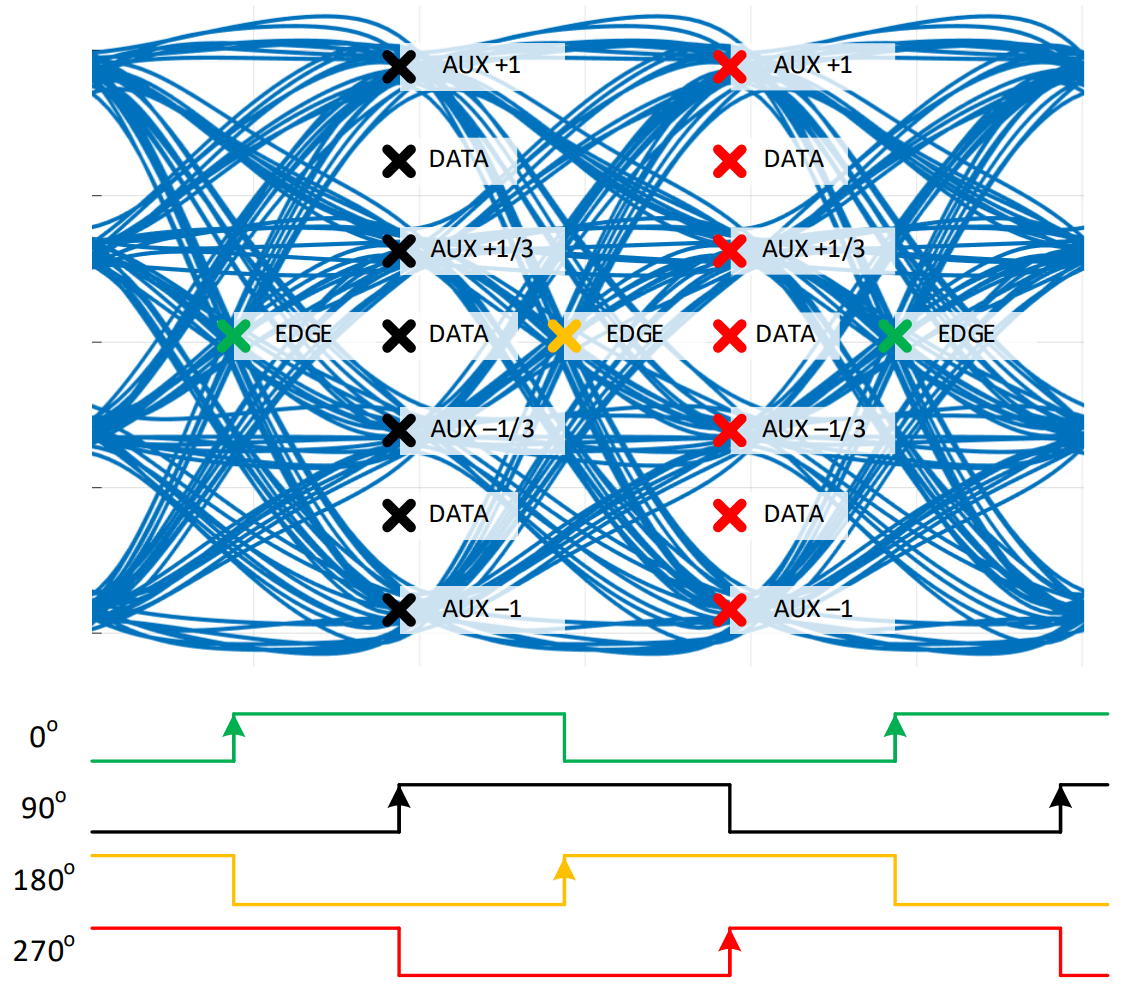Observe the two eyes in each eye diagram of the odd and even summing node waveform outputs. Note that one eye is sharp and one is blurry illustrating that every other eye in each branch is a "don't care" state and allows for the relaxed timing constraint. The IBIS-AMI standard assumes a single-summing node model so to make the dual-summing node model IBIS-AMI compliant, the odd and even waveforms are mux'ed together to create a virtual single-node output waveform.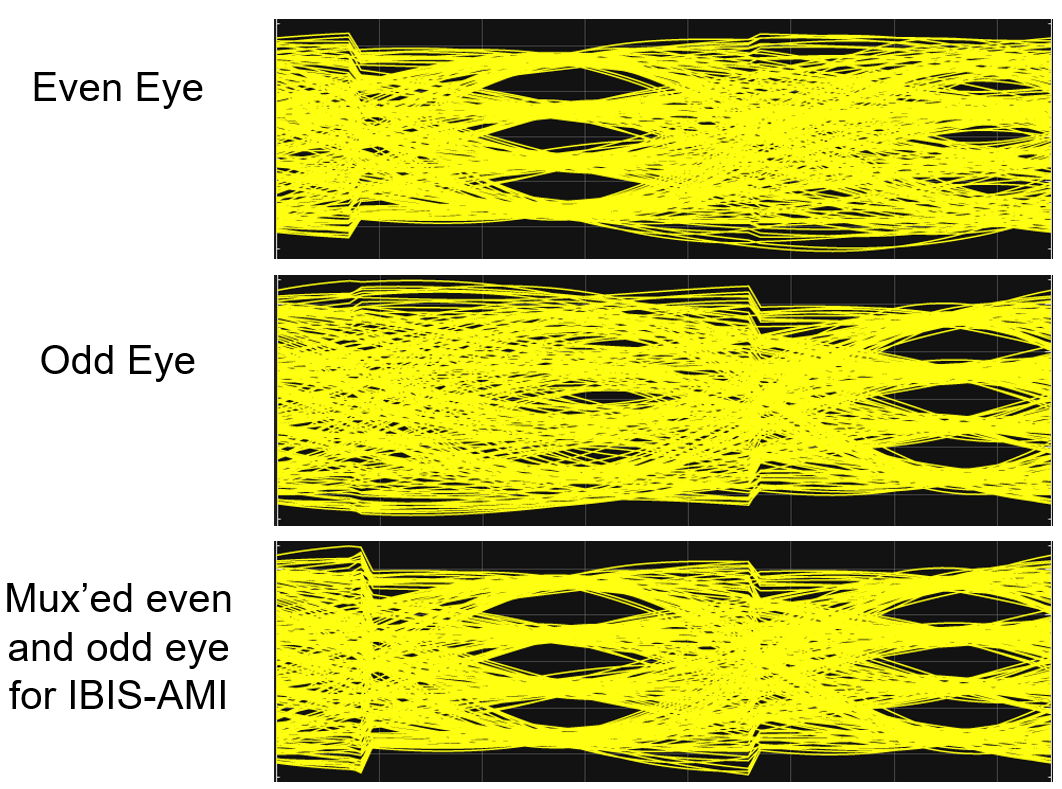With the data path accurately represented, the adaptive behavior of the system can be modeled in detail and utilized for in-depth studies of loop dynamics. The model contains three adaptive loops, 1) clock phase recovery, 2) voltage reference threshold estimation for the samplers, and 3) DFE tap weights. It is critical that the loops converge in the order of clock phase, then voltage references, then DFE taps for optimal performance as shown. The common bang-bang clock phase detector is utilized to determine if the edge samples are early or late. This signal is accumulated and smoothed by the loop filter which in turn drives the voltage-controlled oscillator (VCO), providing the half-rate clocks throughout the model.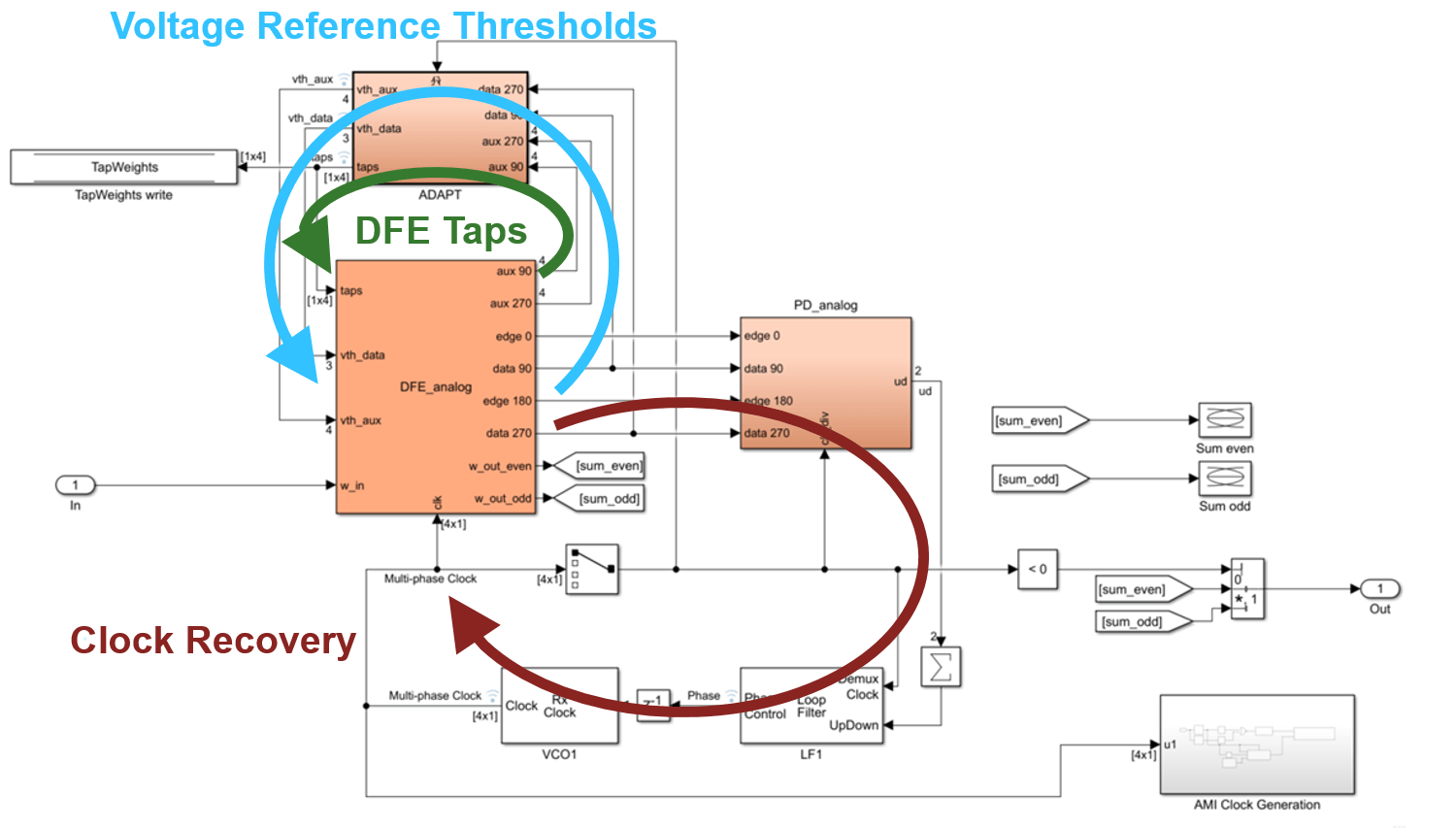Both the DFE tap weight and reference voltage thresholds are estimated with the sign-sign least-mean-square (SS-LMS) algorithm. An LMS algorithm works by observing an input signal and an error signal. By assuming that the system noise is a combination of both random noise and systematic noise, the LMS algorithm searches for this correlated systematic noise between the input and error signal. For the DFE tap estimation, the systematic noise is the channel's residual inter-symbol-interference (ISI) due to loss and reflections and for the reference voltage threshold estimation, the systematic noise is the offset between data and auxiliary samplers. These adaptive filters continually update their weight estimates proportionally to the detected correlation. The sign-sign LMS algorithm is utilized in this model since the output of the signal samplers is binary.

As the DFE tap weight adaptation is simplest to explain it is addressed first, followed by the voltage threshold adaptation. For clock recovery adaptation, see Model Clock Recovery Loops in SerDes Toolbox

#### DFE Tap Weight Adaptation

The 4 tap DFE estimates and compensates for the residual channel ISI. This architectural model is unique in that the LMS algorithm is implemented in both the analog domain, when the DFE taps are applied to the signal and the digital domain when the DFE taps are updated with the LMS Update block. The governing equation for the tap weight update is given below.

`${\mathit{W}}_{\mathit{k}}\left[\mathit{n}\right]={\mathit{W}}_{\mathit{k}}\left[\mathit{n}-1\right]+\mu \text{\hspace{0.17em}}\left(\mathit{D}\left[\mathit{n}-\mathit{k}\right]\cdot {\mathit{E}}_{\mathit{m}}\left[\mathit{n}\right]\cdot \mathit{D}\left[\mathit{n}\right]==\mathit{m}\right)$`

Where

• ${\mathit{W}}_{\mathit{k}}\left[\mathit{n}\right]$ is the tap weight at time sample index $\mathit{n}$ for the ${\mathit{k}}^{\mathrm{th}}$ tap weight (1, 2, 3 or 4).

• $\mu \text{\hspace{0.17em}}$is the adaptation step-size.

• $\mathit{D}\left[\mathit{n}\right]$ is the data symbol value, having the values of -1, -1/3, 1/3 or 1 for PAM4.

• ${\mathit{E}}_{\mathit{m}}\left[\mathit{n}\right]$ is the sign of the error, -1 or 1.

• $\mathit{m}$ is the signal constellation level, having the values of -1, -1/3, 1/3 or 1 for PAM4.

This portion of the model is shown below followed by the view of the 4 tap weights adapting over the course of a simulation.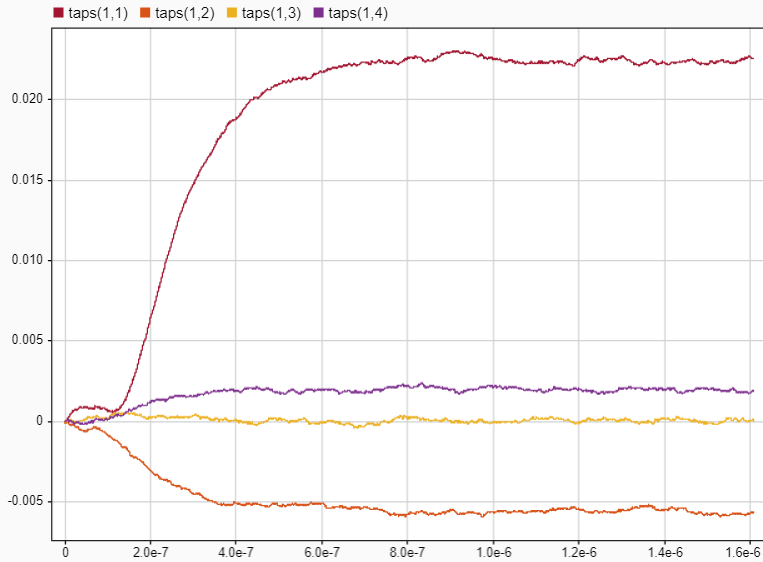#### Voltage Threshold Adaptation

The signal constellation reference voltages for the auxiliary samplers are estimated with a sign-sign LMS algorithm and used to derive the PAM4 data sampler voltage thresholds. Conceptually, when the reference voltage for the auxiliary samplers are converged to the signal constellation values, the auxiliary samplers will on average return an equal number of logic high and low outputs. If the auxiliary reference voltage differs significantly from the signal constellation value, then the average of the output of the sampler will be biased. This correlated bias is used by the LMS algorithm to push the auxiliary reference voltage to converge to the signal constellation voltage. The average value of the adjacent signal constellation voltage estimates is used to derive the data sampler thresholds. The governing equation for the voltage threshold adaption is given below:

`${\mathit{V}}_{{\mathrm{AUX}}_{\mathit{m}}}\left[\mathit{n}\right]={\mathit{V}}_{{\mathrm{AUX}}_{\mathit{m}}}\left[\mathit{n}-1\right]+\mu \cdot \left(1\right)\cdot {\mathit{E}}_{\mathit{m}}\left[\mathit{n}\right]\cdot \left(\mathit{D}\left[\mathit{n}\right]==\mathit{m}\right)$`

Where,

• ${\mathit{V}}_{{\mathrm{AUX}}_{\mathit{m}}}\left[\mathit{n}\right]$ is the auxiliary sample reference voltage for symbol $\mathit{m}$.

• ${\mathit{E}}_{\mathit{m}}\left[\mathit{n}\right]$ is the sign of the error (-1 or 1)

• $\mu \text{\hspace{0.17em}}$is the adaption step size

• $\mathit{n}$ is the sample index

• $\mathit{m}$ is the constellation level

This portion of the model is shown below followed by an example of the startup adaptation of the voltage threshold of the auxiliary samplers ("vth_aux") and the voltage threshold of the data samplers ("vth_data").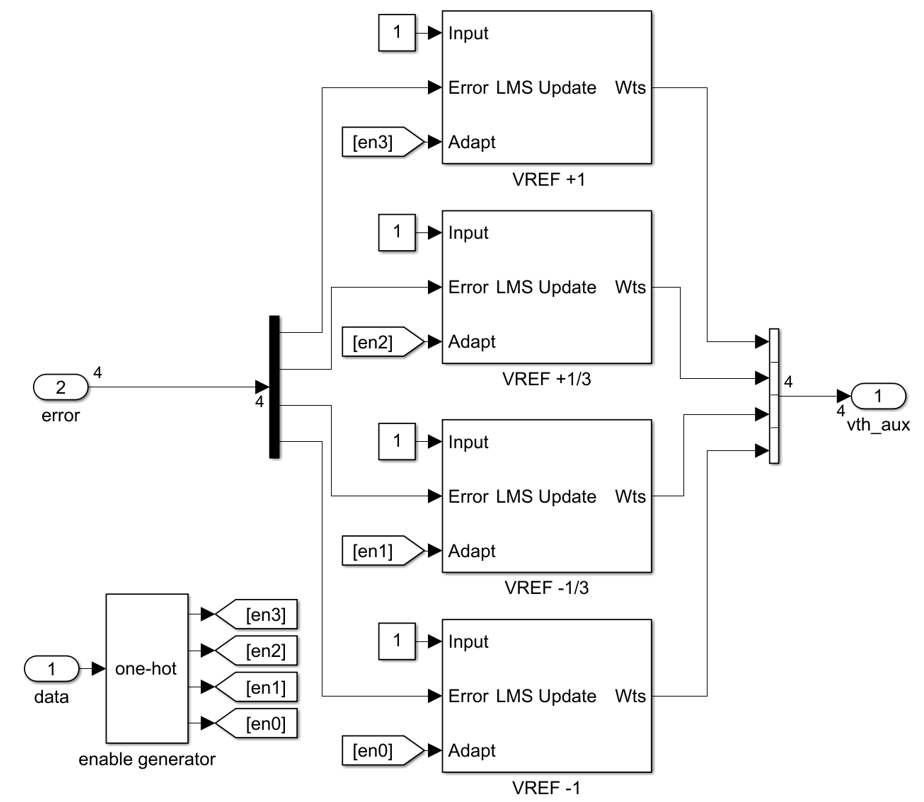### Experiment with Adaptation Loop Convergence

For optimal system performance, it is critical that the adaptive loops converge in the reliable sequence of clock phase first, voltage reference second and DFE tap weights third. The clock phase convergence time is controlled by the loop filter block parameters, up/down counter limit and step size for phase increment/decrement. The DFE tap weight adaptation is controlled by the MATLAB workspace variable, "`muTaps`", which is referenced as the adaptation update step size in the LMS Update block. The voltage threshold adaptation is controlled by the MATLAB workspace varible, "`muThresholds`" which is also referenced as the adaptation update step size in its LMS Update block. These $\mu \text{\hspace{0.17em}}$terms control how much weight to give the adaptation update value versus the existing value. Large values of $\mu \text{\hspace{0.17em}}$will result in faster convergence time but larger steady-state variation and smaller values of $\mu \text{\hspace{0.17em}}$will take longer to converge but have smaller steady-state variation. The determination of what values of $\mu \text{\hspace{0.17em}}$to use for each adaptation loop at the various stages of system operation (startup vs maintenance) is a topic of serious architectural investigation.

The base line behavior of the system with `muTaps` $={2}^{-18\text{\hspace{0.17em}}}$ and `muThresholds`$={2}^{-12}$ is: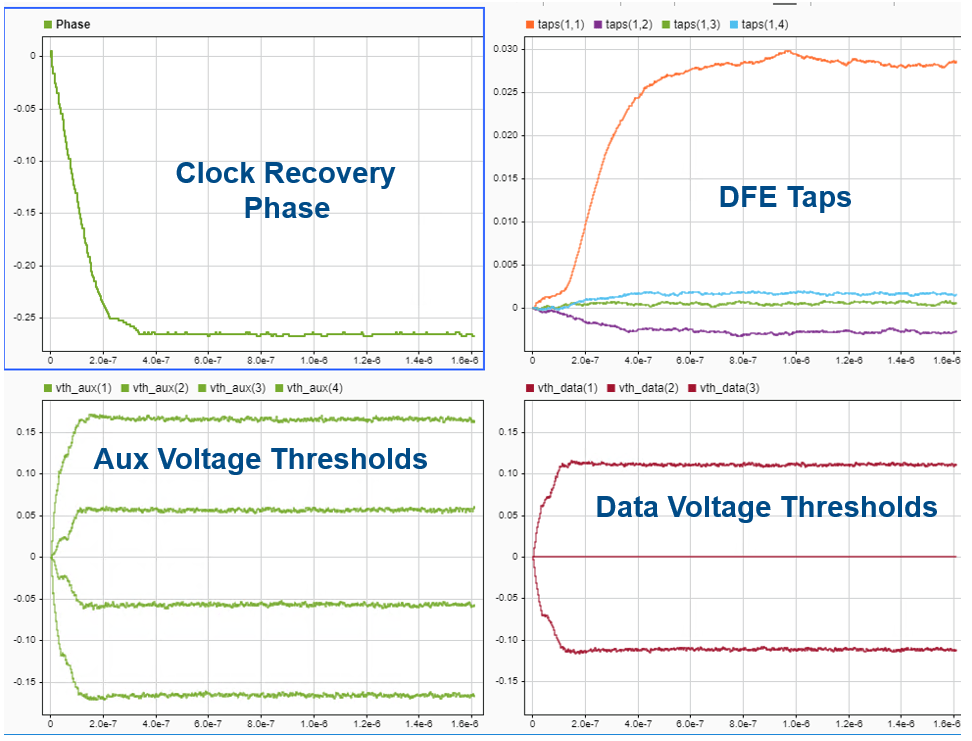If the adaptive loops update is reversed where the DFE tap weights are allowed to converge before the voltage references then unstable behavior is observed. Below is the behavior of the system with `muTaps` $={2}^{-10\text{\hspace{0.17em}}}$ and `muThresholds` $={2}^{-16}$. The updated responses as shown in light blue. Observe how the DFE tap weights vary widely until the voltage thresholds converge. This underscores the need to correctly model and control the numerous adaptive loops in a system.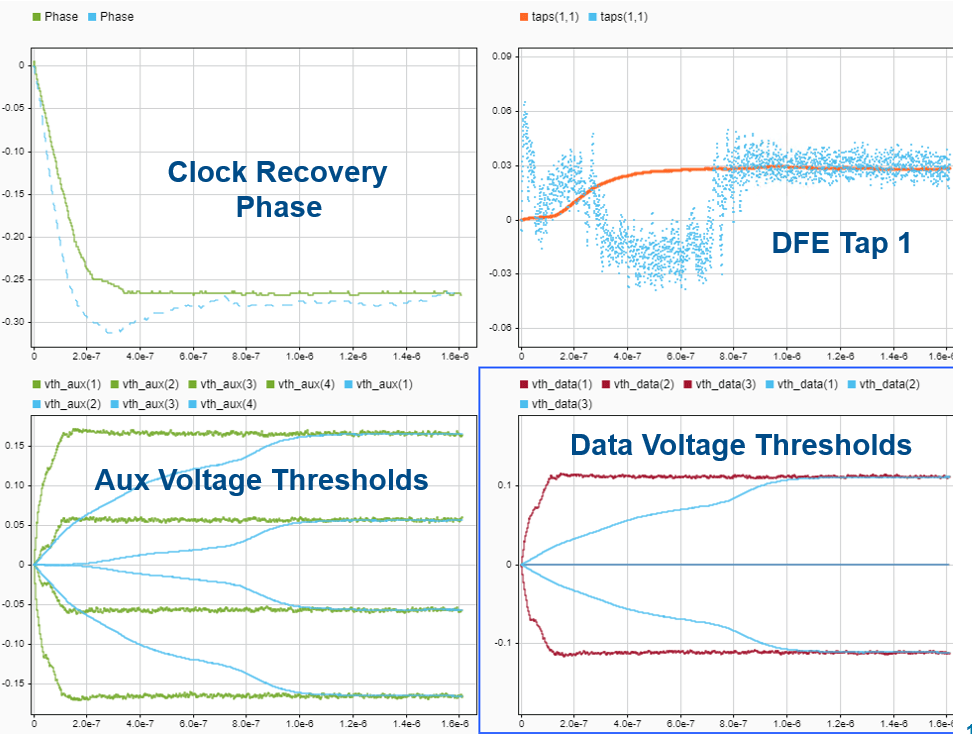### Export to IBIS-AMI and Serial Link Designer

As this model was created within the SerDes Toolbox IBIS-AMI workflow, it is ready to export to AMI and simulated in the Serial Link Designer app.

### Conclusion

Due to decreasing design margins, the increasing difficulty in meeting timing requirements, and difficulty of routing high-speed clocks, a single summing node architectural topology has fundamental limits. This example shows an architecturally representative dual-summing-node-DFE PAM4 receiver model which provides access to realize differences between summing node offsets, bandwidths and timing. The use of modular blocks for the DFE, adaptation engine, phase detector, loop filter and VCO allows for the collaboration between multiple teams and with the advantages of utilizing block interfaces for correlation and verification activities. The representative data path allows for a representative adaptation engine model and the exploration between interactions of the adaptation loops for the clock phase, DFE tap weights and PAM4 threshold voltages.

### References

 S. Ibrahim and B. Razavi, "Low-Power CMOS Equalizer Design for 20-Gb/s Systems," in IEEE Journal of Solid-State Circuits, vol. 46, no. 6, pp. 1321-1336, June 2011, doi: 10.1109/JSSC.2011.2134450.